# Blaschke product

(diff) ← Older revision | Latest revision (diff) | Newer revision → (diff)

Blaschke function

A regular analytic function of a complex variable, defined in the unit discby the finite or infinite product(*)

whereis a non-negative integer, and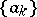,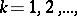is a sequence of points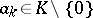such that the product on the right-hand side of (*) converges (the convergence condition is necessary only for an infinite product). The Blaschke product was introduced by W. Blaschke , who proved the following theorem: A sequenceof pointsdefines a function of the type (*) if and only if the seriesis convergent. Each factor of the formcalled the Blaschke factor for, defines a univalent conformal mapping of the disconto itself, which takes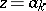to zero, with the normalization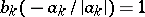. The factors of the form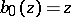may be interpreted as Blaschke factors which correspond to the zerowith the normalization. The definition of Blaschke factors and Blaschke products is readily carried over to a disc of arbitrary radius, and also to an arbitrary simply-connected domain, which is conformally equivalent to a disc.

The sequence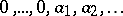(withzeros), which is usually written out in non-decreasing order of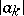, is the sequence of all zeros of the Blaschke product (*) (each zero is written down as many times as its multiplicity). Thus, Blaschke's theorem describes the sequences of zeros of all possible Blaschke products. The product (*) can be regarded as the simplest bounded holomorphic function in the discwith a prescribed sequence of zeros. It converges absolutely and uniformly inside, represents a bounded holomorphic function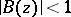in, with angular boundary values of modulus one almost everywhere on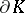. A necessary and sufficient condition for a bounded holomorphic function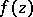in,, to be a Blaschke product, isBlaschke products may be used to give a product representation of important classes of holomorphic functions in the unit disc. Thus, a proof was given for the following theorem of Blaschke: A sequenceof points in the discis the sequence of all zeros of some bounded holomorphic function,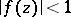, inif and only if the series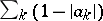is convergent. Moreover,can be represented as a product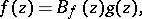whereis the Blaschke product constructed with the zerosof the function, while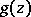is a zero-free holomorphic function in,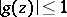, which can be represented relatively simply using an integral formula. Apart from the bounded functions, similar product representations may be constructed for functions of bounded form and for Hardy classes  (cf. Function of bounded form).

The above theory was considerably generalized by M.M. Dzhrbashyan , , who constructed infinite products of a more general nature, which are suitable for the factorization of much larger classes of meromorphic functions. A solution was also found for the problem of constructing analogues of Blaschke products and Blaschke's theorem for doubly-connected domains  and, in general, finitely-connected  domains. The solution of the problem of constructing suitable analogues of the Blaschke product for holomorphic functions of several complex variables is rendered very difficult by the fact that the zeros of such functions cannot be isolated.

How to Cite This Entry:
Blaschke product. Encyclopedia of Mathematics. URL: http://encyclopediaofmath.org/index.php?title=Blaschke_product&oldid=11306
This article was adapted from an original article by P.M. Tamrazov (originator), which appeared in Encyclopedia of Mathematics - ISBN 1402006098. See original article